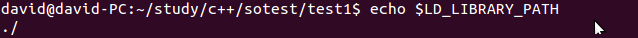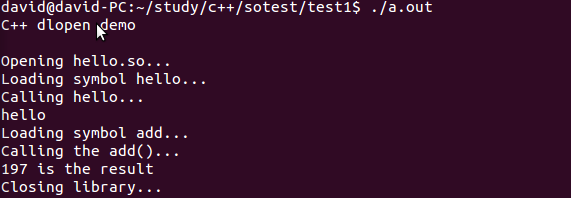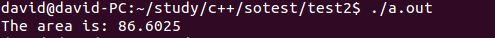# C++调用自己的.so

1. dlopen加载c++ 函数及类： http://blog.csdn.net/lwj1396/article/details/5204484

3. 动态调用动态库方法 .so: http://blog.csdn.net/lbmygf/article/details/7401862

### 然后从介绍Name Mangling开始

C++有个特定的关键字用来声明采用C binding的函数：extern “C” 。 用 extern “C”声明的函数将使用函数名作符号名，就像C函数一样。因此，只有非成员函数才能被声明为extern “C”，并且不能被重载。尽管限制多多，extern “C”函数还是非常有用，因为它们可以象C函数一样被dlopen动态加载。冠以extern “C”限定符后，并不意味着函数中无法使用C++代码了，相反，它仍然是一个完全的C++函数，可以使用任何C++特性和各种类型的参数。

### 示例程序1. 加载简单函数main.cpp的代码如下

[cpp]
//———-
//main.cpp:
//———-
#include
#include

int main() {
using std::cout;
using std::cerr;

cout << "C++ dlopen demo\n\n";

// open the library
cout << "Opening hello.so…\n";
void* handle = dlopen("libhello.so", RTLD_LAZY);

if (!handle) {
cerr << "Cannot open library: " << dlerror() << ‘\n’;
return 1;
}

typedef void (*hello_t)();

// reset errors
dlerror();
hello_t hello = (hello_t) dlsym(handle, "hello");
const char *dlsym_error = dlerror();
if (dlsym_error) {
cerr << "Cannot load symbol ‘hello’: " << dlsym_error <<
‘\n’;
dlclose(handle);
return 1;
}

// use it to do the calculation
cout << "Calling hello…\n";
hello();

// reset errors
dlerror();
if (dlsym_error) {
‘\n’;
dlclose(handle);
return 1;
}

// use it to do the calculation
cout << add(98, 99) << " is the result\n";

// close the library
cout << "Closing library…\n";
dlclose(handle);
}

[/cpp]

[cpp]
//———-
// hello.cpp:
//———-
#include

extern "C" void hello() {
std::cout << "hello" << ‘\n’;
}

extern "C" int add(int a, int b){
return a + b;
}
[/cpp]

1)       dlopen

a.根据环境变量LD_LIBRARY_PATH查找

b.根据/etc/ld.so.cache查找

c.查找依次在/lib和/usr/lib目录查找。

flag参数表示处理未定义函数的方式，可以使用RTLD_LAZY或RTLD_NOW。RTLD_LAZY表示暂时不去处理未定义函数，先把库装载到内存，等用到没定义的函数再说；RTLD_NOW表示马上检查是否存在未定义的函数，若存在，则dlopen以失败告终。

2)       dlerror

3)       dlsym

4)       dlclose

\$ g++ -shared -fPIC -o libhello.so hello.cpp
\$ g++ main.cpp -ldl### 示例程序2. 加载类

———————————-polygon.hpp—————————————————————

[cpp]
//———-
//polygon.hpp:
//———-
#ifndef POLYGON_HPP
#define POLYGON_HPP

class polygon {
protected:
double side_length_;

public:
polygon()
: side_length_(0) {}

virtual ~polygon() {}

void set_side_length(double side_length) {
side_length_ = side_length;
}

virtual double area() const = 0;
};

// the types of the class factories
typedef polygon* create_t();
typedef void destroy_t(polygon*);

#endif

[/cpp]

=0表示这个成员函数是纯虚函数，也就是它可以没有定义，只有接口，由它的继承类具体定义它的行为，当然，你也可以给它定义缺省的函数体

create_t* create_triangle = (create_t*) dlsym(triangle, “create”);

[cpp]
[/cpp]

———————————————-main.cpp———————————————-

[cpp]
//———-
//main.cpp:
//———-
#include "polygon.hpp"
#include
#include

int main() {
using std::cout;
using std::cerr;

void* triangle = dlopen("triangle.so", RTLD_LAZY);
if (!triangle) {
cerr << "Cannot load library: " << dlerror() << ‘\n’;
return 1;
}

// reset errors
dlerror();

create_t* create_triangle = (create_t*) dlsym(triangle, "create");
const char* dlsym_error = dlerror();
if (dlsym_error) {
cerr << "Cannot load symbol create: " << dlsym_error << ‘\n’;
return 1;
}

destroy_t* destroy_triangle = (destroy_t*) dlsym(triangle, "destroy");
dlsym_error = dlerror();
if (dlsym_error) {
cerr << "Cannot load symbol destroy: " << dlsym_error << ‘\n’;
return 1;
}

// create an instance of the class
polygon* poly = create_triangle();

// use the class
poly->set_side_length(10);
cout << "The area is: " << poly->area() << ‘\n’;

// destroy the class
destroy_triangle(poly);

dlclose(triangle);
}
[/cpp]

———————————————-triangle.cpp———————————————-

[cpp]
//———-
//triangle.cpp:
//———-
#include "polygon.hpp"
#include

class triangle : public polygon {
public:
virtual double area() const {
return side_length_ * side_length_ * sqrt(3) / 2;
}
};

// the class factories
extern "C" polygon* create() {
return new triangle;
}

extern "C" void destroy(polygon* p) {
delete p;
}

[/cpp]

go on..

\$ g++ -shared -fPIC -o triangle.so triangle.cpp
\$ g++ main.cpp -ldl
\$ ./a.out## 3 Replies to “C++调用自己的.so”

1.google authorship code says:

When some one searches for his necessary thing, therefore
he/she wants to be available that in detail, therefore that thing
is maintained over here.

2.Anonymous says:

mm

1.David says:

WTF

This site uses Akismet to reduce spam. Learn how your comment data is processed.